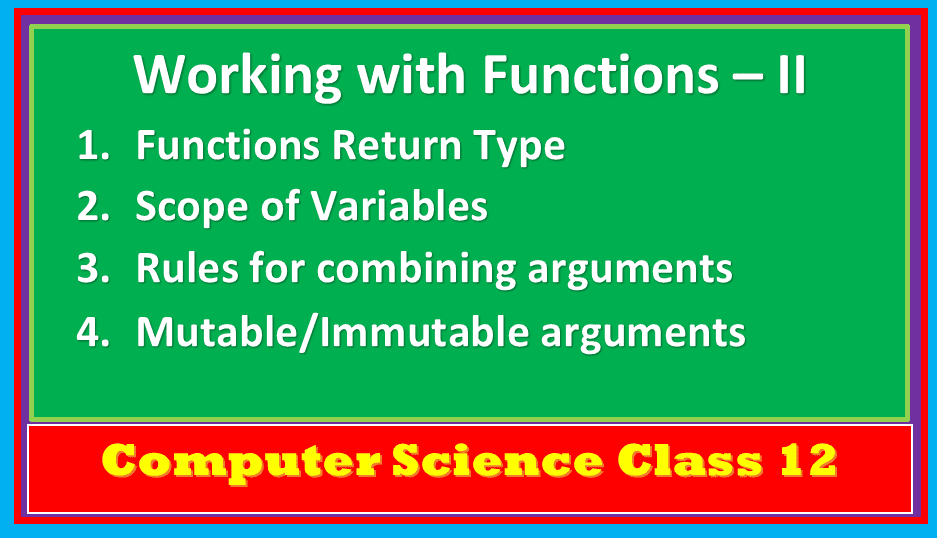Python functions class12 provides you the detailed notes about function types, return values, and scope of the function variables.

Topics Covered

## Python functions class12- function types

Python supports three types of functions:

• Built in Functions: Pre-defined functions of python such as len(), type(), input() etc.
• Functions defined in modules: Function defined in particular modules, can be used when module is imported. Module is a container of functions, variables, constants, class in a separate file which can be reused. The module can be imported in two ways
1. import statement: Used to import the entire module. EX. import math
2. from statement : import all functions or the selected one. EX. from random import randint
• User Defined Functions: Function created by the programmer

## User-Defined Functions – Python functions class12

In above program, two function created i) simple_interest() and main() and executed. simple_interest() function receives three parameters p,r and n. main() function prompts to input these values and accepted as an arguments in calling simple_interest() function.

## Parameters and Arguments in Function

Parameters are the values provided at the time of function definition. For Ex. p,r and n.

Arguments are the values passed while calling a function. For Ex. princ_amt, r, n in main().

Types of Arguments:

Python supports four argument types:

1. Positional Arguments: Arguments passed to a function in correct positional order, no. of arguments must match with no. of parameters required.
2. Default Arguments: Assign default to value to a certain parameter, it is used when the user knows the value of the parameter, default values are specified in the function header. It is optional in the function call statement. If not provided in the function call statement then the default value is considered. Default arguments must be provided from right to left.
3. Key Word Arguments: Keyword arguments are the named arguments with assigned values being passed in function call statement, the user can combine any type of argument.
4. Variable Length Arguments: It allows the user to pass as many arguments as required in the program. Variable-length arguments are defined with * symbol.
``````def sum(*n):
total=0
for i in n:
total+=i
print(“Sum = “, total)
sum()
# Calling function
sum()  # o/p  sum=0
sum(10) #o/p  sum=10
sum(10,20,30,40) #o/p sum=100``````

## Rules for combining three types of arguments

1. An argument list must contain positional arguments followed by any keyword argument.

2. Keyword arguments should be taken from the required arguments preferably.

3. Value of argument can’t be specified more than once.

Ex.:

cal_discount(amt=500,rate=10) or cal_discount(rate=10,amt=500)

## Python functions class12-Returning values from function

A function in python may or may not return a value. A function does not return a values is called void function. A result will be printed in same function.

A function which returns a value is a function that displays the calculated result. The value returned to a specific variable. The result will be printed in some other function.

## Python functions class12 – Scope of Variable

A variable scope refers to access location of a variable.

There are two kinds of scopes:

Global Variable: A variable that is declared in top level statements is called global variable. To access the value of global variable user need to write global keyword in front of variable in a function.

Local Variable: A name declared in specific function body is called local variable.

## Mutable / immutable of arguments / parameters & function calls

All variables in python stores the memory address of the actual object not value itself. Although mutable and immutable objects and variables handled differently during working with function arguments.

Immutable objects are those object whose values cannot be changed. They allocate new memory whenever value is changed.

New memory allocated for x when value assigned x=45. Every time when value is changed memory address also gets changed. When new object is created old object will be discarded and memory cells make free for use.

### Passing mutable type value (List)

As you know list, dictionary etc are mutable data types in python.

### Passing Strings:

Consider following program:

Thank you fore reading the article. Share your valuable feedback in comment section.

## Checkout More

Computer Science
Informatics Practice

Check out python functions documentations.

1.raj says:
2.tutorialaicsip.com says: